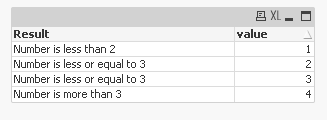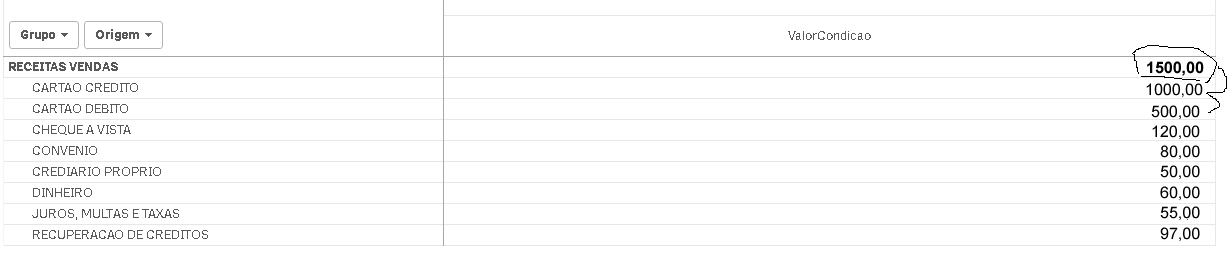# New to Qlik Sense

Discussion board where members can get started with Qlik Sense.

cancel
Showing results for
Did you mean:
HighlightedNot applicable

## Calculated measure with several conditions

I need to create a calculated measure with several conditions. Theoretically need to implement as an expression:

if (condition) then

else if (condition) then

else

I could only implement the If and Else.

Tags (1)
8 Replies
HighlightedMVP

## Re: Calculated measure with several conditions

The nested if isn't working?

If(Condition1Met, Something1,

If(Condition2Met, Something2,

If(Condition3Met, Something3.....

HighlightedSpecialist III

## Re: Calculated measure with several conditions

Give us more details.

As Sunny said use Nested Ifs.

For example:

```LOAD *,
if(value < 2, 'Number is less than 2',
If(value <= 3, 'Number is less or equal to 3', 'Number is more than 3')) as Result
INLINE [
value
1
2
3
4
];
```

The outcome would be this:Practically you can you as many nested ifs as you wish. But according to the situation you can apply other methods to reach the result.

HighlightedNot applicable

## Re: Calculated measure with several conditions

but I needed to do the calculation of expressions because they need to calculate only a few lines of the PivotTable.

HighlightedNot applicable

## Re: Calculated measure with several conditions

I can use within expressions? And how I use Else?

HighlightedSpecialist III

## Re: Calculated measure with several conditions

If statement can be used in the script and in the expression. In both cases the logic is the same.

If works like following:

IF(logical condition, if logical condition is TRUE then do something, if logical condition is FALSE then do something)

Please test and create few examples yourself and you could easily handle the issue. Or just attach the sample qvw file.MVP

## Re: Calculated measure with several conditions

I think if you can provide a sample with your expected output, we might be able to offer you better help rather than some guessworkHighlightedNot applicable

## Re: Calculated measure with several conditions

Because the goal is a few lines is the sum of a few lines below as in the image:HighlightedCreator II

## Re: Calculated measure with several conditions

Hi Thiago,

Have you ever been able to get the answer for your question?

LD